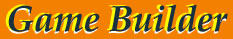# Free VB ebook

Automation Word - game forms builder Version 1.00 and Visual Basic eBook
(c) by Valery V Shmeleff Moscow/Russia www.oflameron.ru and www.oflameron.comTCP and Winsock (Ebook)Free eBookTemplatesWeb DesignFree hostingWallpapers

This ebook shows how to use OLE automation by creating and editing MS Office Word document. If you are a beginner and you want to
know more about OLE, then you should read this ebook.View my Forum
PDF Images Gallery
Hi Tech News
 Using printable game as promotion tools. (Translated from the newspaper)     Mr G., a news agent, developed and introduced a simple promotion tool to boost sales. ...He had a series of large-format paper game forms printed, to put into the newspapers that were bought from him. In a month, the news agent's total sales grew by 14 per cent. The sales were up not only for the newspapers supplemented with paper game forms, but for other products that were sold at the agent's, too.
 About this game. If you are going on vacation with children, take some copies of this table game with you “Oflameron”. You should not pay money for it – it is free of charge. Just download the file with the game forms in a popular Word format from the site and print the necessary number of forms. You children will be engaged in a catching contest and you can have a good rest. The game “Oflameron” is not like other games you already know. 1, 2, 3 or 4 players can play on one blank (game form).     If you would like to take part in the game you would have a good time as well. The game is interesting both for adults and for children. The aim of the game is to increase a personal account. The person who has got the highest score wins. It provides for one more feature of the game – it can be gambling. If you give a price to each point you can hold “financial” contest with you partner. But be attentive please. By the end of the game the difference in points can amount to several thousand. Thus you can lose or win a considerable amount of money. The game can be a good solution for an advertising campaign. If you have a business of your own you can place your advertisement on the form. Be sure – all players will see it more than once!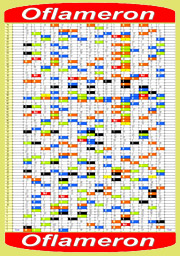game form screenshort[ vb programmer's tutorial ]Free print-and-play game PDF

## Free VB ebook

Game Form.Builder A programme for automatic generation and duplex printing of printable paper game Oflameron - Game Form.Builder The program Game Form.Builder uses the functions of MS Office Word software to generate game forms in a Word document format and enables duplex printing of the finished game form using a printer. One side of the sheet will have a table with a game field printed. The reverse side will feature rules, examples and clues. The program has two operation modes: "full" (slow) and "partial" (fast). The full mode generates a game from scratch. It involves extensive computations, formatting and redesign. It may take as long as 5 minutes to produce a game form in a full mode. The partial mode only recalculates a part of the game field (approximately 15 per cent). This makes it possible to very quickly produce a new game version and print a desired number of copies. A generated game form (an MS Word document) may be edited manually any way you want it. For example, it can be supplemented with sponsor advertisements. A finished game form document can be saved, emailed or printed in any number of copies.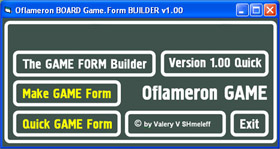Game Form.Builder is a programme (freeware) that is designed for automatic generation and printing of printable game form. All you have to do is
download a ZIP file with the program (1.6 Mb), unzip it and start the installer (setup.exe) Just answer the installer's questions and select the options you need. The generated game form can be saved in the MS Word format, edited or printed in the required number of copies. The game form is to be printed out on an A4 sheet of paper on both sides, with one side used for the game grid and the reverse, for clues, rules, an example and recommendations. The program has been tested with Microsoft Office Word 2003 under OS Windows 2000 and Windows XP

### Free VB ebook

[ VB forum, submit code ] :: [ VB articles ] :: [ VB tutorial ] :: [ Game ]- generation of a completely original game form based on a template (Word document in the XML format). The values of all 960 cells of the game grid are assigned by a random number generator. Since generation takes place using the MS Word software, it is relatively slow. It takes approximately 5 minutes to complete an original game table. The completed form remains open in the Word processor. It can be edited, for example, supplemented with a sponsor advert, saved on a disc, printed in any number of copies, etc.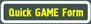- fast generation of a completely original game form (game table) based on a template (Word document in the XML format). For a form to be quickly put together, the values of 4 bottom levels only are generated. Nevertheless, this proves sufficient to produce a game table which for all intents and purposes will be original. Illustration of the process of game form generation. Source Code on Visual Basic 6.0        VB CODE DOWNLOAD >>    TUTORIAL DOWNLOAD >>          Dim wrd As New Word.Application ''Create Word as COM    [ web1 ] [ web2 ] [ web3 ] [ web4 ] [ vb ]     Dim Pt As String, St As String ''Declare variables          Private Sub Picture2_Click() ''Standard generation of game formSt = App.Path ''Path to generator program         St = St + "\oflameron-form2.xml" ''Path to XML template         wrd.Visible = True ''Open Word         Set doc = wrd.Documents.Add(St) ''Add Word doc         Dim k, g         For i = 0 To 15           For j = 0 To 59           Randomize ''Use random number generator           k = Int((20 * Rnd) + 1)           g = g + 1           frmOflameron.Caption = "Complete " + CStr(g) + " cells from 960"    [ Java ebook ] [ Free tutorials ]                      If k = 1 Then repltext = "1"           If k = 1 Then FntColor = wdColorBlack ''Symbol color           If k = 1 Then CellColor = wdColorWhite ''Cell background color     +1                        If k = 2 Then repltext = "-1"           If k = 2 Then FntColor = wdColorBlack ''Symbol color           If k = 2 Then CellColor = wdColorWhite ''Cell background color     -1                        If k = 3 Then repltext = "5"           If k = 3 Then FntColor = wdColorBlack ''Symbol color           If k = 3 Then CellColor = wdColorWhite ''Cell background color     +5                        If k = 4 Then repltext = "-5"           If k = 4 Then FntColor = wdColorBlack ''Symbol color           If k = 4 Then CellColor = wdColorWhite ''Cell background color     -5
 If k = 5 Then repltext = "+10"           If k = 5 Then FntColor = wdColorBlack ''Symbol color           If k = 5 Then CellColor = wdColorWhite ''Cell background color     +10                        If k = 6 Then repltext = "-10"           If k = 6 Then FntColor = wdColorBlack ''Symbol color           If k = 6 Then CellColor = wdColorWhite ''Cell background color     -10                        If k = 7 Then repltext = "+15"           If k = 7 Then FntColor = wdColorBlack ''Symbol color           If k = 7 Then CellColor = wdColorWhite ''Cell background color     +15                        If k = 8 Then repltext = "-15"           If k = 8 Then FntColor = wdColorBlack ''Symbol color           If k = 8 Then CellColor = wdColorWhite ''Cell background color     -15                        If k = 9 Then repltext = "25"           If k = 9 Then FntColor = wdColorBlack ''Symbol color           If k = 9 Then CellColor = wdColorWhite ''Cell background color     25                        If k = 10 Then repltext = "T"           If k = 10 Then FntColor = wdColorWhite ''Symbol color           If k = 10 Then CellColor = wdColorSeaGreen ''Cell background color      T                         If k = 11 Then repltext = "-25"           If k = 11 Then FntColor = wdColorBlack ''Symbol color           If k = 11 Then CellColor = wdColorWhite ''Cell background color     -25                        If k = 12 Then repltext = "P"           If k = 12 Then FntColor = wdColorBlack ''Symbol color           If k = 12 Then CellColor = wdColorLightBlue ''Cell background color      P                         If k = 13 Then repltext = "B"           If k = 13 Then FntColor = wdColorBlack ''Symbol color           If k = 13 Then CellColor = wdColorLightYellow ''Cell background color      B                         If k = 14 Then repltext = "Z"           If k = 14 Then FntColor = wdColorWhite ''Symbol color           If k = 14 Then CellColor = wdColorBlack ''Cell background color      Z                         If k = 15 Then repltext = "Z"           If k = 15 Then FntColor = wdColorWhite ''Symbol color           If k = 15 Then CellColor = wdColorBlack ''Cell background color      Z                         If k = 16 Then repltext = "End"           If k = 16 Then FntColor = wdColorWhite ''Symbol color           If k = 16 Then CellColor = wdColorRed ''Cell background color    End                       If k = 17 Then repltext = "-10"           If k = 17 Then FntColor = wdColorBlack ''Symbol color           If k = 17 Then CellColor = wdColorWhite ''Cell background color     -10                        If k = 18 Then repltext = "-5"           If k = 18 Then FntColor = wdColorBlack ''Symbol color           If k = 18 Then CellColor = wdColorWhite ''Cell background color     -5

If k = 19 Then repltext = "-1"
If k = 19 Then FntColor = wdColorBlack ''Symbol color
If k = 19 Then CellColor = wdColorWhite ''Cell background color     -1

If k = 20 Then repltext = "+1"
If k = 20 Then FntColor = wdColorBlack ''Symbol color
If k = 20 Then CellColor = wdColorWhite ''Cell background color     +1

If k = 21 Then repltext = "+5"
If k = 21 Then FntColor = wdColorBlack ''Symbol color
If k = 21 Then CellColor = wdColorWhite ''Cell background color     +5
'=============================
wrd.ActiveWindow.Selection.Find.Text = "sh" ''Find text “sh” on game form
wrd.ActiveWindow.Selection.Find.Replacement.Text = repltext ''Replace text "sh" with "T"
wrd.ActiveWindow.Selection.Find.Wrap = wdFindContinue ''Continue search
wrd.ActiveWindow.Selection.Find.Execute Replace:=wdReplaceOne ''Replace

wrd.ActiveWindow.Selection.SelectCell ''Highlight the entire cell with the text replaced with “T”
wrd.ActiveWindow.Selection.Font.Color = FntColor ''“T” symbol color
wrd.ActiveWindow.Selection.Cells.Shading.BackgroundPatternColor = CellColor ''Replace the colour of the highlighted cell

Next j
Next i

wrd.ActiveDocument.PrintOut Copies:=1, Pages:="1,2", ManualDuplexPrint:=False ''Duplex printing of pages 1 and 2

End Sub

## FreeVBebook

Private Sub Picture3_Click() ''Quick mode''Fast generation of game form
Pt = App.Path ''Path to generator program
Pt = Pt + "\oflameron-form2quick.xml" ''Path to XML template
wrd.Visible = True ''Open Word
Set doc = wrd.Documents.Add(Pt) ''Add Word doc
Dim k, g
g = 896
For i = 0 To 15
For j = 0 To 3   ''Levels 0-3 only !!!!
Randomize ''Use random number generator
k = Int((20 * Rnd) + 1)
g = g + 1
frmOflameron.Caption = "Complete " + CStr(g) + " cells from 960"

If k = 1 Then repltext = "1"
If k = 1 Then FntColor = wdColorBlack ''Symbol color
If k = 1 Then CellColor = wdColorWhite ''Cell background color     +1

If k = 2 Then repltext = "-1"
If k = 2 Then FntColor = wdColorBlack ''Symbol color
If k = 2 Then CellColor = wdColorWhite ''Cell background color     -1

If k = 3 Then repltext = "5"
If k = 3 Then FntColor = wdColorBlack ''Symbol color
If k = 3 Then CellColor = wdColorWhite ''Cell background color     +5

If k = 4 Then repltext = "-5"
If k = 4 Then FntColor = wdColorBlack ''Symbol color
If k = 4 Then CellColor = wdColorWhite ''Cell background color     -5

 If k = 5 Then repltext = "+10"           If k = 5 Then FntColor = wdColorBlack ''Symbol color           If k = 5 Then CellColor = wdColorWhite ''Cell background color     +10                        If k = 6 Then repltext = "-10"           If k = 6 Then FntColor = wdColorBlack ''Symbol color           If k = 6 Then CellColor = wdColorWhite ''Cell background color     -10                        If k = 7 Then repltext = "+15"           If k = 7 Then FntColor = wdColorBlack ''Symbol color           If k = 7 Then CellColor = wdColorWhite ''Cell background color     +15                        If k = 8 Then repltext = "-15"           If k = 8 Then FntColor = wdColorBlack ''Symbol color           If k = 8 Then CellColor = wdColorWhite ''Cell background color     -15                        If k = 9 Then repltext = "25"           If k = 9 Then FntColor = wdColorBlack ''Symbol color           If k = 9 Then CellColor = wdColorWhite ''Cell background color     25                        If k = 10 Then repltext = "T"           If k = 10 Then FntColor = wdColorWhite ''Symbol color           If k = 10 Then CellColor = wdColorSeaGreen ''Cell background color      T                         If k = 11 Then repltext = "-25"           If k = 11 Then FntColor = wdColorBlack ''Symbol color           If k = 11 Then CellColor = wdColorWhite ''Cell background color     -25                        If k = 12 Then repltext = "P"           If k = 12 Then FntColor = wdColorBlack ''Symbol color           If k = 12 Then CellColor = wdColorLightBlue ''Cell background color      P                         If k = 13 Then repltext = "B"           If k = 13 Then FntColor = wdColorBlack ''Symbol color           If k = 13 Then CellColor = wdColorLightYellow ''Cell background color      B                         If k = 14 Then repltext = "Z"           If k = 14 Then FntColor = wdColorWhite ''Symbol color           If k = 14 Then CellColor = wdColorBlack ''Cell background color      Z                         If k = 15 Then repltext = "Z"           If k = 15 Then FntColor = wdColorWhite ''Symbol color           If k = 15 Then CellColor = wdColorBlack ''Cell background color      Z                         If k = 16 Then repltext = "End"           If k = 16 Then FntColor = wdColorWhite ''Symbol color           If k = 16 Then CellColor = wdColorRed ''Cell background color    End                       If k = 17 Then repltext = "-10"           If k = 17 Then FntColor = wdColorBlack ''Symbol color           If k = 17 Then CellColor = wdColorWhite ''Cell background color     -10                        If k = 18 Then repltext = "-5"           If k = 18 Then FntColor = wdColorBlack ''Symbol color           If k = 18 Then CellColor = wdColorWhite ''Cell background color     -5

If k = 19 Then repltext = "-1"
If k = 19 Then FntColor = wdColorBlack ''Symbol color
If k = 19 Then CellColor = wdColorWhite ''Cell background color     -1

If k = 20 Then repltext = "+1"
If k = 20 Then FntColor = wdColorBlack ''Symbol color
If k = 20 Then CellColor = wdColorWhite ''Cell background color     +1

If k = 21 Then repltext = "+5"
If k = 21 Then FntColor = wdColorBlack ''Symbol color
If k = 21 Then CellColor = wdColorWhite ''Cell background color     +5
'=============================
wrd.ActiveWindow.Selection.Find.Text = "sh" ''Find text “sh” on game form
wrd.ActiveWindow.Selection.Find.Replacement.Text = repltext ''Replace text "sh" with "T"
wrd.ActiveWindow.Selection.Find.Wrap = wdFindContinue ''Continue search
wrd.ActiveWindow.Selection.Find.Execute Replace:=wdReplaceOne ''Replace

wrd.ActiveWindow.Selection.SelectCell ''Highlight the entire cell with the text replaced with “T”
wrd.ActiveWindow.Selection.Font.Color = FntColor ''“T” symbol color
wrd.ActiveWindow.Selection.Cells.Shading.BackgroundPatternColor = CellColor ''Replace the colour of the highlighted cell

Next j
Next i

wrd.ActiveDocument.PrintOut Copies:=1, Pages:="1,2", ManualDuplexPrint:=False ''Duplex printing of pages 1 and 2

End Sub

Private Sub Picture4_Click()
ShellExecute hwnd, "open", "http://word.oflameron.ru", vbNullString, vbNullString, conSwNo
End Sub

Private Sub Picture5_Click()
ShellExecute hwnd, "open", "http://www.oflameron.com", vbNullString, vbNullString, conSwNo
End Sub

Private Sub Picture6_Click()
ShellExecute hwnd, "open", "http://delphi.oflameron.com", vbNullString, vbNullString, conSwNo
End Sub

Private Sub Picture7_Click()
End
End Sub

## FreeVBebook

The program uses MS Word features: VB |Project| -> |References| -> and select "Microsoft Word 11.0 Object Library" if you use Microsoft Word 2003 (or "Microsoft Word 9.0 Object Library" and etc)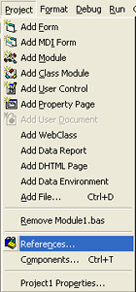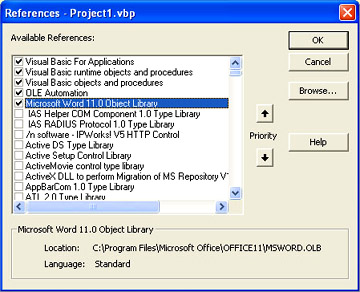Load VB 6.0 Project: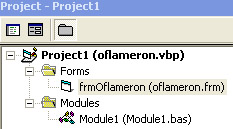The path to XML template as:                    St = App.Path           St = St + "\oflameron-form2.xml"                    for EXE Builder application (!)                             For use Builder from VB 6.0 the correct path is: Flashcards Images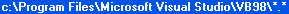Or: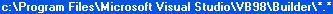Delphi Tutorial  Free step-by-step tutorial in ebook format  Free ebook         Homemade game  Barbie  Netvouz bookmarks page  Homemade game  Free VB ebook  Free game  Free games  Barbie  Oflameron  Visual Basic  Download flashcards  Flashcards free  Printable flashcard  Free download  Flashcards  Äîě  Ńŕěîäĺëüíűĺ čăđű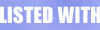ee@oflameron.ru

PDF Images. Free Visual Basic ebook. Free VB ebook. PDF ebook. Oflameron freeware
Flashcards example